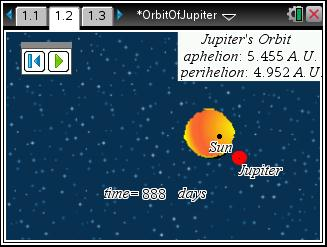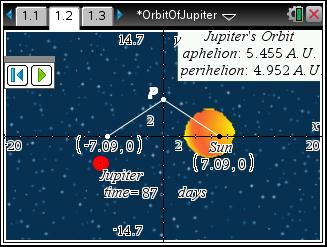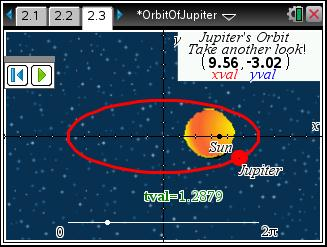# Activities

••• ##### Subject Area

• Math: Algebra II: Conics

• ##### Author9-12

45 Minutes

• ##### Device
•TI-Nspire™ CX/CX II
•TI-Nspire™ CX CAS/CX II CAS
• TI-Nspire™ Navigator™
• TI-Nspire™
• TI-Nspire™ CAS
• ##### Software

TI-Nspire™
TI-Nspire™ CAS

3.2

• ##### Other Materials
By Lynne Plettenberg

## Orbit Of Jupiter

#### Activity Overview

This activity explores models for the elliptical orbit of Jupiter. Problem 1 reviews the geometric definition of an ellipse as students calculate a and b from the perihelion and aphelion of Jupiter. Problem 2 develops the concept of a parametric curve by using a data capture to discover the coordinate equations of an ellipse.

#### Key Steps

•In Problem 1, students use information about Jupiter’s orbit to form an equation and model. Students study an animated model of the planet’s orbit. They should see that the orbit is elliptical, with the sun at one focus of the ellipse.

•They use their observations and the information given to build a Cartesian Model. To assist them, they use the general equation of an ellipse centered at (0, 0).

•In Problem 2, students get the opportunity to introduce parametric equations. This is necessary since this ellipse cannot be expressed as a single equation in terms of only x and y. Instead, x and y are defined in terms of t as follows x = f(t) and y = g(t).